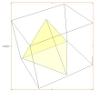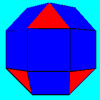#### You may also like### A Mean Tetrahedron

Can you number the vertices, edges and faces of a tetrahedron so that the number on each edge is the mean of the numbers on the adjacent vertices and the mean of the numbers on the adjacent faces?### Rhombicubocts

Each of these solids is made up with 3 squares and a triangle around each vertex. Each has a total of 18 square faces and 8 faces that are equilateral triangles. How many faces, edges and vertices does each solid have?### Icosian Game

This problem is about investigating whether it is possible to start at one vertex of a platonic solid and visit every other vertex once only returning to the vertex you started at.

# Which Solids Can We Make?

##### Age 11 to 14Challenge Level

Sometimes it helps to think about two dimensions to get a better understanding of what happens in three dimensions:

In two dimensions, the interior angles of convex polygons are always less than $180^{\circ}$. Can you explain why?
In three dimensions, the sum of the interior angles at each vertex of convex polyhedra must be less than $360^{\circ}$. Can you explain why?

The exterior angles of polygons are a measure of how far short the angles are from $180^{\circ}$.
The angle deficit at a vertex of a polyhedron is a measure of how far short each angle sum is from $360^{\circ}$.

The sum of the exterior angles of a polygon is always $360^{\circ}$.
What do you notice about the total angle deficit of a solid?
This table might help:

 Angle Sum Angle Deficit Number of Vertices Total Angle Deficit Cube 270 90 8 720 Tetrahedron Octahedron Icosahedron Dodecahedron